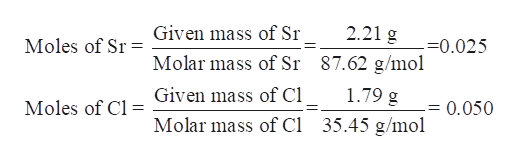# If 2.21 grams of strontium combines with 1.79 grams of chlorine to form a chloride, calculate the percent composition of the compound.

Question
6 views

If 2.21 grams of strontium combines with 1.79 grams of chlorine to form a chloride, calculate the percent composition of the compound.

check_circle

Step 1

If 2.21 grams of strontium combines with 1.79 grams of chlorine to form a chloride, the percent composition of the compound is to be calculated.

Step 2

Write the chemical equation of reaction between strontium and chlorine-

Strontium and chlorine reacts to form strontium chloride (SrCl2).

Step 3

Determine the moles of each reactant present.

Mass of Sr = 2.21 g

Molar mass of Sr = 87...help_outlineImage TranscriptioncloseGiven mass of Sr 2.21 g Moles of Sr -=0.025 87.62 g/mol Molar mass of Sr 1.79 g Given mass of Cl Moles of Cl 0.050 35.45 g/mol Molar mass of Cl fullscreen

### Want to see the full answer?

See Solution

#### Want to see this answer and more?

Solutions are written by subject experts who are available 24/7. Questions are typically answered within 1 hour.*

See Solution
*Response times may vary by subject and question.
Tagged in

### General Chemistry